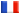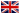# Séminaire de Cryptographie

## Eimar Byrne### An Algebraic Decoding of Negacyclic Codes Over the Integers Modulo 4

In the 1960s, Berlekamp introduced the negacyclic codes over GF(p) and described an efficient decoder that corrects any t Lee errors, where p > 2t. We consider this family of codes, defined over the integers modulo 4. We show that if a generator polynomial for a Z4 negacyclic code C has roots a^{2j+1} for j=0,...,t, where a is a primitive 2n th root of unity in a Galois extension of Z4, then C is a t Lee error-correcting code. We present a corresponding decoding algorithm that corrects any t Lee errors. The treatment given here uses techniques from Groebner bases, although this is not essential to the decoding method.